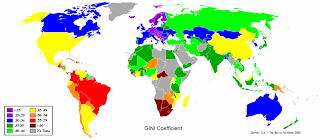## Thursday, March 1, 2012

### Gini coefficientThe Gini coefficient (also known as the Gini index or Gini ratio) is a measure of statistical dispersion developed by the Italian statistician and sociologist Corrado Gini and published in his 1912 paper "Variability and Mutability" (Italian: Variabilità e mutabilità).
The Gini coefficient measures the inequality among values of a frequency distribution (for example levels of income). A Gini coefficient of zero expresses perfect equality where all values are the same (for example, where everyone has an exactly equal income). A Gini coefficient of one (100 on the percentile scale) expresses maximal inequality among values (for example where only one person has all the income).
It has found application in the study of inequalities in disciplines as diverse as sociology, economics, health science, ecology, chemistry, engineering and agriculture. It is commonly used as a measure of inequality of income or wealth. Worldwide, Gini coefficients for income range from approximately 0.23 (Sweden) to 0.70 (Namibia) although not every country has been assessed.